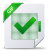# On the optimality of the Hedge algorithm in the stochastic regime

On the optimality of the Hedge algorithm in the stochastic regime

Introduction

Our contribution

This work fills a gap in the existing literature by providing an analysis of the standard Hedge algorithm in the stochastic setting. We show that the anytime Hedge algorithm with default learning rate ηt ∝ p log(M)/t actually adapts to the stochastic setting, in which it achieves an optimal constant O(log(M)/∆) regret bound without any dedicated tuning for the easier instance, which might be surprising at first sight. This contrasts with previous works, which require the construction of new adaptive (and more involved) algorithms. Remarkably, this property is not shared by the variant of Hedge for a known fixed-horizon T with constant learning rate η ∝ p log(M)/T, since it suffers a Θ(√ T log M) regret even in  easier instances. This exhibits a strong difference between the performances of the anytime and the fixed-horizon variants of the Hedge algorithm. Given the aforementioned adaptivity of Decreasing Hedge, one may wonder whether there is in fact any benefit in using more sophisticated algorithms in the stochastic regime. We answer this question affirmatively, by considering a more refined measure of complexity of a stochastic instance than the gap ∆. Specifically, we show that Decreasing Hedge does not admit improved regret under Bernstein conditions, which are standard low-noise conditions from statistical learning (Mammen and Tsybakov, 1999; Tsybakov, 2004; Bartlett and Mendelson, 2006). By contrast, it was shown by Koolen et al. (2016) that algorithms which satisfy some adaptive adversarial regret bound achieve improved regret under Bernstein conditions. Finally, we characterize the behavior of Decreasing Hedge in the stochastic regime, by showing that its eventual regret on any stochastic instance is governed by the gap ∆.

Lire sur cLicours.com :  Les propriétés du lait de chamelle

Related work

In the bandit setting, where the feedback only consists of the loss of the selected action, there has also been some interest in “best-of-both-worlds” algorithms that combine optimal O( √ MT) worst-case regret in the adversarial regime with improved O(M log T) regret (up to logarithmic factors) in the stochastic case (Bubeck and Slivkins, 2012; Seldin and Slivkins, 2014; Auer and Chiang, 2016). In particular, Seldin and Slivkins (2014); Seldin and Lugosi (2017) showed that by augmenting the standard EXP3 algorithm for the adversarial regime (an analogue of Hedge with Θ(1/ √ t) learning rate) with a special-purpose gap detection mechanism, one can achieve poly-logarithmic regret in the stochastic case. This result is strengthened in some recent follow-up work (Zimmert and Seldin, 2019; Zimmert et al., 2019), which appeared since the completion of the first version of the present work, that obtains optimal regret in the stochastic and adversarial regimes through a variant of the Follow-The-Regularized-Leader (FTRL) algorithm with Θ(1/ √ t) learning rate and a proper regularizer choice. This result can be seen as an analogue in the bandit case of our upper bound for Decreasing Hedge. On the other hand, in the bandit setting, the hardness of an instance is essentially characterized by the gap ∆ (Bubeck and Cesa-Bianchi, 2012); in particular, the Bernstein condition, which depends on the correlations between the losses of the experts, cannot be exploited under bandit feedback, where one only observes one arm at each round. Hence, it appears that the negative part of our results (on the limitations of Hedge) does not have an analogue in the bandit case. A similar adaptivity result for FTRL with decreasing Θ(1/ √ t) learning rate has been observed in a different context by Huang et al. (2017). Specifically, it is shown that, in the case of online linear optimization on a Euclidean ball, FTRL with squared norm regularizer and learning rate Θ(1/ √ t) achieves O(log T) regret when the loss vectors are i.i.d. This result is an analogue of our upper bound for Hedge, since this algorithm corresponds to FTRL on the simplex with entropic regularizer (Cesa-Bianchi and Lugosi, 2006; Hazan, 2016). On the other hand, the simplex lacks the curvature of the Euclidean ball, which is important to achieve small regret; here, the improved regret is ensured by a condition on the distribution, namely the existence of a gap ∆. Our lower bound for Hedge shows that this condition is necessary, thereby characterizing the long-term regret of FTRL on the simplex with entropic regularizer. In the case of the Euclidean ball with squared norm regularizer, the norm of the expected loss vector appears to play a similar role, as shown by the upper bound from Huang et al. (2017).  Outline. We define the setting of prediction with expert advice and the Hedge algorithm in Section 4.2, and we recall herein its standard worst-case regret bound. In Section 4.3, we consider the behavior of the Hedge algorithm on easier instances, namely the stochastic setting with a gap ∆ on the best expert. Under an i.i.d assumption on the sequence of losses, we provide in Theorem 4.1 an upper bound on the regret of order (log M)/∆ for Decreasing Hedge. In Proposition 4.2, we prove that the rate (log M)/∆ cannot be improved in this setting. In Theorem 4.2 and Corollary 4.1, we extend the regret guarantees to the adversarial with a gap setting, where a leading expert linearly outperforms the others. These results stand for any Hedge algorithm which is worst-case optimal and with any learning rate which is larger than the one of Decreasing Hedge, namely O( p log M/t). In Proposition 4.3, we prove the sub-optimality of the fixed-horizon Hedge algorithm, and of another version of Hedge based on the so-called “doubling trick”. In Section 4.4, we discuss the advantages of adaptive Hedge algorithms, and explain what the limitations of Decreasing Hedge are compared to such versions. We include numerical illustrations of our theoretical findings in Section 4.5, conclude in Section 4.6 and provide the proofs in Section 4.7.Télécharger le document complet